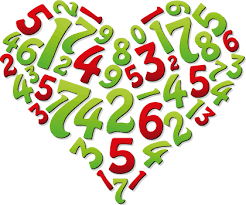Q) What is the next number in the series 01, 1011, 111021, 31101211…… ?

Q) In a peach orchard, each harvested fruit is assigned a category based on its weight. A regular peach weighs 200 gms while a premium peach weighs 250 gms. A worker accidentally put one of the baskets containing premium peaches on the regular peach shelf. All peaches look the same. How can you identify the premium peach basket in a single weighing?

Q) If abcd x 4 = dcba. What is the value of a+b+c+d?

• What is the solution?

By Akhilesh Poddar, 4 weeks ago

• In the island of Hoola Boola Moola , inhabitants have a...

By Sanket211, 3 months ago

• For a=0.9086, x= 1.0286 and a= -1.6627, x= 4.9173

By Aditya Dang, 4 months ago

• Since, x+1 satisfies the equation ax^2+ bx+ c=0. Theref...

By Aditya Dang, 4 months ago

• The HCF of 141 and 517 is 47. But 47 does not completel...

By Aditya Dang, 4 months ago

• 1. How many integral pairs (x, y), where 0< x, y <...

By lastknight99, 4 months ago

• Hi, It is given that a= (212)b . Forming an equation...

By Aditya Dang, 4 months ago

• Perimeter of circle is 2pi(793)^1/2.

By Aditya Dang, 4 months ago

• Area of ΔDOC is 21cm^2.

By Aditya Dang, 4 months ago

• Length of smaller of two sides is equal to 36 units.

By Aditya Dang, 4 months ago

• Length of AB is (17)^1/2 units.

By Aditya Dang, 4 months ago

• The sum borrowed is Rs. 3300.

By Aditya Dang, 4 months ago